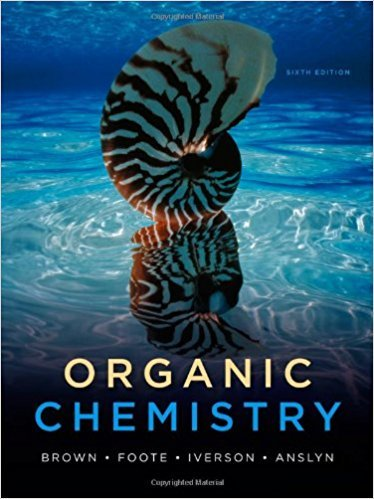×
×

# Each compound gives only one signal in its 1 H-NMRISBN: 9780840054982 88

## Solution for problem 13.3 Chapter 13

Organic Chemistry | 6th Edition

• Textbook Solutions
• 2901 Step-by-step solutions solved by professors and subject experts
• Get 24/7 help from StudySoup virtual teaching assistantsOrganic Chemistry | 6th Edition

4 5 1 273 Reviews
15
1
Problem 13.3

Each compound gives only one signal in its 1 H-NMR spectrum. Propose a structural formula for each compound. (a) C3H6O (b) C5H10 (c) C5H12 (d) C4H6Cl4

Step-by-Step Solution:
Step 1 of 3

2 D= ρV S∙(C +k∙C ) 2 1ρV S∙k∙C...

Step 2 of 3

Step 3 of 3

##### ISBN: 9780840054982

Since the solution to 13.3 from 13 chapter was answered, more than 330 students have viewed the full step-by-step answer. Organic Chemistry was written by and is associated to the ISBN: 9780840054982. This full solution covers the following key subjects: compound, NMR, gives, its, formula. This expansive textbook survival guide covers 29 chapters, and 1350 solutions. The answer to “Each compound gives only one signal in its 1 H-NMR spectrum. Propose a structural formula for each compound. (a) C3H6O (b) C5H10 (c) C5H12 (d) C4H6Cl4” is broken down into a number of easy to follow steps, and 26 words. This textbook survival guide was created for the textbook: Organic Chemistry, edition: 6. The full step-by-step solution to problem: 13.3 from chapter: 13 was answered by , our top Chemistry solution expert on 09/09/17, 04:06AM.

Unlock Textbook Solution